# Non Verbal Reasoning - Analogy

### Exercise :: Analogy - Section 2

Each of the following questions consists of two sets of figures. Figures A, B, C and D constitute the Problem Set while figures 1, 2, 3, 4 and 5 constitute the Answer Set. There is a definite relationship between figures A and B. Establish a similar relationship between figures C and D by selecting a suitable figure from the Answer Set that would replace the question mark (?) in fig. (D).

6.

Select a suitable figure from the Answer Figures that would replace the question mark (?).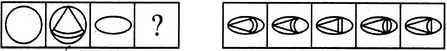(A)     (B)      (C)     (D)                  (1)      (2)      (3)      (4)      (5)

 A. 1 B. 2 C. 3 D. 4 E. 5

Explanation:

A cone is introduced inside the figure. The arcs in the cone are parts of the main figure.

7.

Select a suitable figure from the Answer Figures that would replace the question mark (?).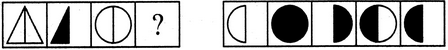(A)     (B)      (C)     (D)                  (1)      (2)      (3)      (4)      (5)

 A. 1 B. 2 C. 3 D. 4 E. 5

Explanation:

The right half of the figure is lost and the remaining portion is shaded.

8.

Select a suitable figure from the Answer Figures that would replace the question mark (?).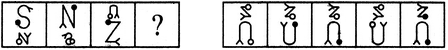(A)     (B)      (C)     (D)                  (1)      (2)      (3)      (4)      (5)

 A. 1 B. 2 C. 3 D. 4 E. 5

Explanation:

The upper element moves to the lower position; changes its size (reduces in size if initially larger and enlarges if initially smaller); gets laterally inverted and its circular part changes colour (becomes black if initially white and vice-versa). The lower element moves to the upper position; changes its size (reduces in size if initially larger and enlarges if initially smaller); rotates through 180o and its circular part changes colour (becomes black if initially white and vice-versa).

9.

Select a suitable figure from the Answer Figures that would replace the question mark (?).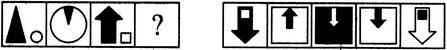(A)     (B)      (C)     (D)                  (1)      (2)      (3)      (4)      (5)

 A. 1 B. 2 C. 3 D. 4 E. 5

Explanation:

The smaller element gets enlarged. The larger element reduces in size; gets vertically inverted; moves inside the other element and gets attached to its upper end.

10.

Select a suitable figure from the Answer Figures that would replace the question mark (?).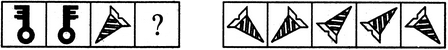(A)     (B)      (C)     (D)                  (1)      (2)      (3)      (4)      (5)

 A. 1 B. 2 C. 3 D. 4 E. 5